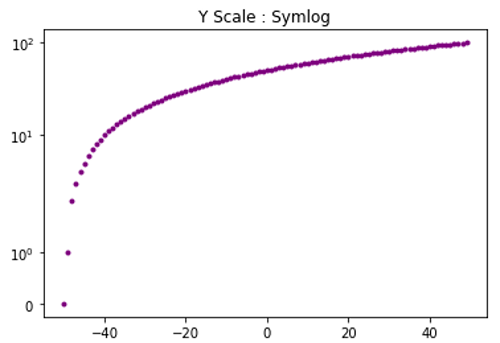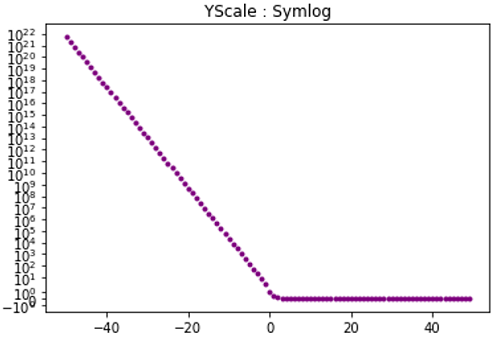# Python | Symmetric Log Scale for Y axis in Matplotlib

In this article, we are going to set y axis scale as symlog for mathematical data visualization.
Submitted by Anuj Singh, on August 14, 2020

When we need to plot data in symmetric logarithmic form, then we can use an inbuilt defined function matplotlib.pyplot.yscale('symlog'). We have illustrated the usage using the below example and also compared it with the linear scale in the subplot.

Illustration:## Python code for symmetric log scale for y axis in matplotlib

```import numpy as np
import matplotlib.pyplot as plt

dt = 1
x = np.arange(-50.0, 50.0, dt)
y = np.arange(0, 100.0, dt)

plt.figure()
plt.plot(x, y, '.', color='purple')
plt.title('Y Scale : Symlog')
plt.yscale('symlog')
plt.show()

y = np.exp(-x)
plt.figure()
plt.plot(x, y, '.', color='purple')
plt.title('YScale : Symlog')
plt.yscale('symlog')
plt.show()
```

Output:

```Output is as Figure
```

Languages: » C » C++ » C++ STL » Java » Data Structure » C#.Net » Android » Kotlin » SQL
Web Technologies: » PHP » Python » JavaScript » CSS » Ajax » Node.js » Web programming/HTML
Solved programs: » C » C++ » DS » Java » C#
Aptitude que. & ans.: » C » C++ » Java » DBMS
Interview que. & ans.: » C » Embedded C » Java » SEO » HR
CS Subjects: » CS Basics » O.S. » Networks » DBMS » Embedded Systems » Cloud Computing
» Machine learning » CS Organizations » Linux » DOS
More: » Articles » Puzzles » News/Updates